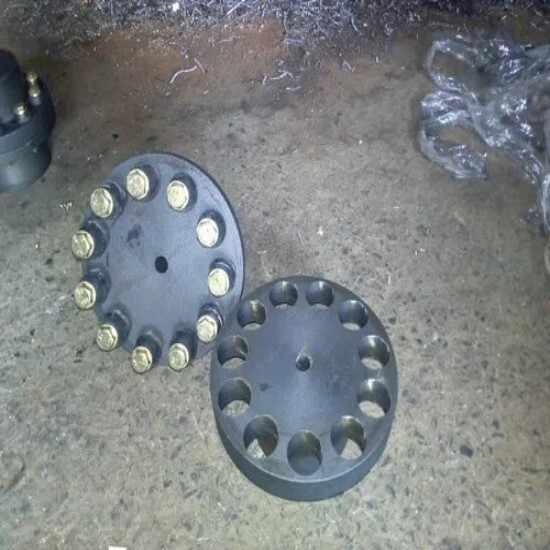0Pin & Bush Type Coupling
Product Description

Pin & Bush Type Coupling:

Simple & Compact in Construction the Coupling is capable of transmitting high torques at maximum speeds. The flanges are manufactured with cast iron, grade 20 of I.S. 210. This type of coupling permits drive in either direction & requires neither lubrication nor adjustment after fitting. The flexible bushes remain unafftected by water, dust & atmospheric condition.
Available in Ex-stock OD - 3"

Specification :

 SIZE RATE SIZE RATE 3" 225=00 12" 4149=00 4" 294=00 13" 6828=00 5" 450=00 14" 7087=00 6" 674=00 15" 8495=00 7" 1073=00 16" 9680=00 8" 1387=00 18" 13478=00 9" 1969=00 20" 20375=00 10" 2665=00 21" 25940=00 11" 3801=00 22" 27940=00 24" 38016=00
Related Products